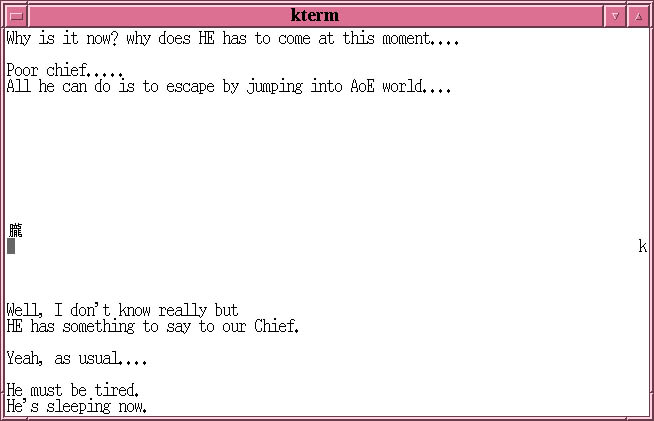Next: 2.5 \$BB>\$N%f!<%6\$H\$N2qOC(B(2) Up: 2. \$B%[%9%H\$H%f!<%6>pJs(B Previous: 2.3 \$B%a%C%;!<%8\$NI=<((B

# 2.4\$BB>\$N%f!<%6\$H\$N2qOC(B(1) -- talk

talk\$B%3%^%s%I\$OB>\$N%f!<%6\$H%j%"%k%?%\$%`\$G2qOC\$r\$9\$k\$?\$a\$N%D!<%k\$G\$"\$j!\$(BCNS\$B\$N\$9\$Y\$F\$N%[%9%H\$GMxMQ\$G\$-\$k!%%?!<%_%J%k%&%#%s%I%&\$r>e2<(B2\$B\$D\$KJ,3d\$7\$F!\$(B2\$B?M\$,=q\$\$\$?J8;z\$rF1;~\$KI=<(\$9\$k5!G=\$r;}\$C\$F\$\$\$k!%F~NO\$7\$?J8;z\$O(B1\$BJ8;z\$:\$DN>talk\$B%3%^%s%I\$G\$OF|K\8l\$,;HMQ\$G\$-\$J\$\$\$N\$G!\$\$R\$i\$,\$J\$K\$h\$kI=<(\$rK>\$`>l9g\$K\$O8e=R\$9\$k(Bphone\$B%3%^%s%I(B\$B\$rMxMQ\$9\$k!%Aje\$N\$I\$3\$N%[%9%H\$G\$"\$C\$F\$b%j%"%k%?%\$%`\$G2qOC\$G\$-\$k!%(B

talk\$B%3%^%s%I\$r\$N8e\$K(B`\$B%m%0%\$%sL>(B@\$B%[%9%HL>(B \$BC(B'\$B\$H\$\$\$&7A\$G2qOC\$r\$9\$kAj\$HF1;~\$K%[%9%HL>\$*\$h\$SC\$r;XDj\$9\$k!%(B

`% talk [\$B%m%0%\$%sL>(B]@[\$B%[%9%HL>(B] [\$BC(B]<RET>`
`% talk s00000hf@ccz00 pts/2<RET>`
talk\$B%3%^%s%I\$re2<\$KJ,3d\$5\$l\$?>uBV\$K\$J\$j!\$AjuBV\$K\$J\$k!%Ajtalk \$BAj(B@\$B%[%9%HL>(B'\$B\$HF~NO\$9\$k!%t00000tf)\$B\$+\$i\$+\$+\$C\$F\$-\$?(Btalk\$B\$K1~\$(\$F2qOC\$r;O\$a\$h\$&\$H\$7\$F\$\$\$k\$H\$3\$m\$G\$"\$k!%(B
```Message from Talk_Daemon@ccz00 at 6:02 ...
talk: connection requested by t00000tf@ccz01
talk: respond with: talk t00000tf@ccz01

% talk t00000tf@ccz01<RET>```
\$B<+J,\$,F~NO\$7\$?J8;z\$O%?!<%_%J%k%&%#%s%I%&\$N>eB&\$K!\$Aj2.1)\$B!%(Btalk\$B\$r=*N;\$9\$k\$K\$O(BC-c\$B\$r2!\$9!%(B

\$B%7%'%k\$+\$i(Bemacs -nw\$B\$G(BEmacs\$B\$r5/F0\$7\$F\$\$\$k>uBV\$N\$H\$-\$K(Btalk\$B\$,\$+\$+\$C\$F\$-\$F\$b!\$\$=\$N\$^\$^\$N>uBV\$G\$O\$9\$0\$K1~\$(\$i\$l\$J\$\$!%\$3\$N\$h\$&\$J>l9g\$K\$O(B1\$BEY(BC-z\$B\$r2!\$7\$F!\$8=:_\$N%3%^%s%I(B(\$B\$3\$3\$G\$O(B emacs -nw)\$B\$r%5%9%Z%s%I\$7\$F\$+\$i(Btalk\$B%3%^%s%I\$rfg\$B%3%^%s%I\$rF~NO\$9\$k\$H!\$%5%9%Z%s%I>uBV\$N%3%^%s%I(B(\$B\$3\$3\$G\$O(Bemacs -nw)\$B\$rI|5"\$G\$-\$k!%%5%9%Z%s%I\$K\$D\$\$\$F\$O!\$(B\$BBh(B1\$BIt(B 2.5.8\$B\$r;2>H\$9\$k\$3\$H!%(BNext: 2.5 \$BB>\$N%f!<%6\$H\$N2qOC(B(2) Up: 2. \$B%[%9%H\$H%f!<%6>pJs(B Previous: 2.3 \$B%a%C%;!<%8\$NI=<((B